Поиск даташита (1.687.043 компонентов) Где искатьв наименованиив описании### Datasheet: M11B11664A-30T (No company)

64 K x 16 DRAM EDO PAGE MODE
Скачать:PDFZIP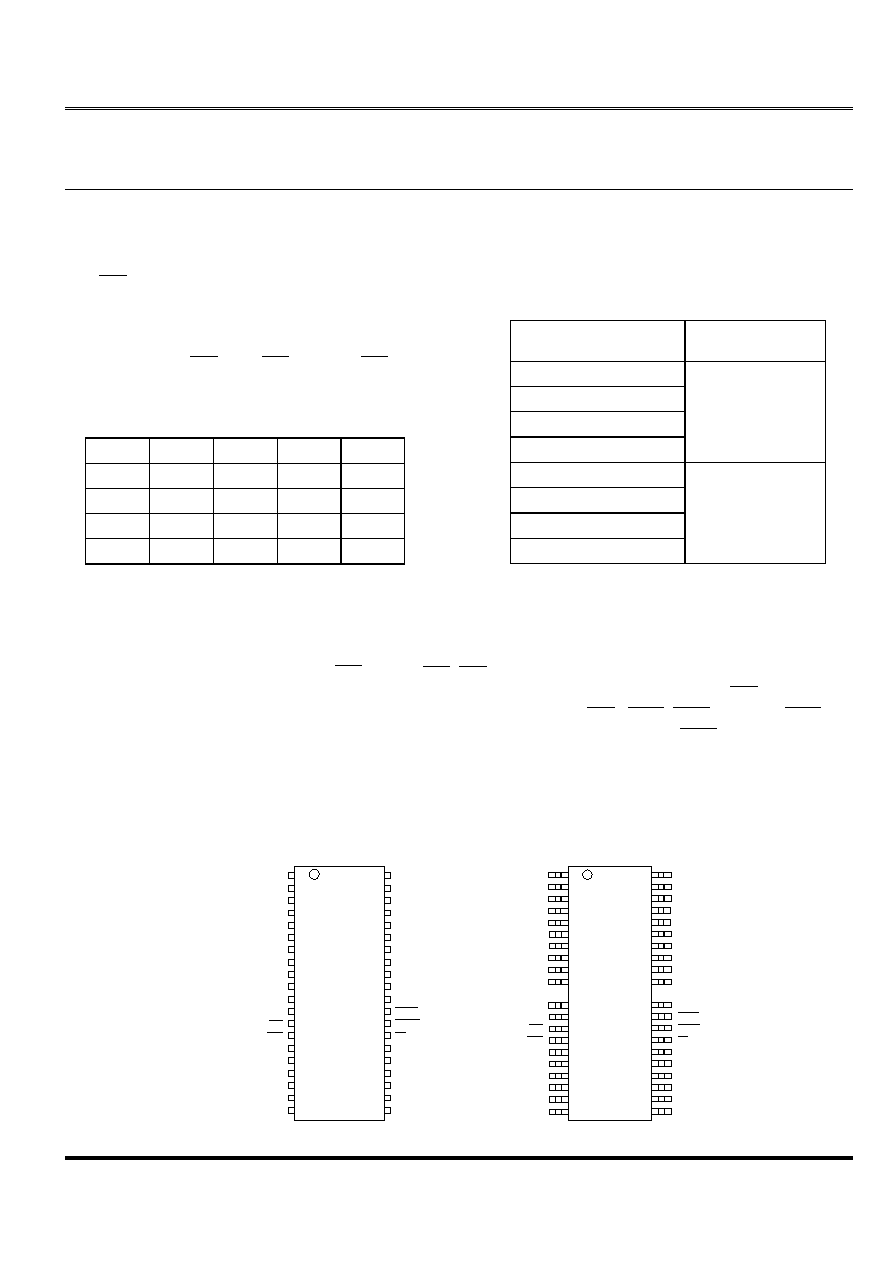(OLWH07
M11B11664A
Elite Memory Technology Inc
Publication Date : Dec. 2000
Revision : 1.3 1/15
DRAM
64 K x 16 DRAM
EDO PAGE MODE
FEATURES
y
X16
organization
y
EDO (Extended Data-Output) access mode
y
y
Single
5V
(
10%) power supply
y
TTL-compatible inputs and outputs
y
256-cycle refresh in 4ms
y
Refresh
modes
: RAS only, CAS BEFORE RAS (CBR)
and HIDDEN
y
JEDEC
standard
pinout
y
Key AC Parameter
t
RAC
t
CAC
t
RC
t
PC
-25
25
8
43
10
-30
30
9
55
12
-35
35
10
65
14
-40
40
11
75
16
ORDERING INFORMATION - PACKAGE
40-pin 400mil SOJ
44 / 40-pin 400mil TSOP (TypeII)
PRODUCT NO.
PACKING TYPE
M11B11664A-25J
M11B11664A-30J
M11B11664A-35J
M11B11664A-40J
SOJ
M11B11664A-25T
M11B11664A-30T
M11B11664A-35T
M11B11664A-40T
TSOPII
GENERAL DESCRIPTION
The M11B11664A is a randomly accessed solid state memory, organized as 65,536 x 16 bits device. It offers Extended
Data-Output , 5V(
10%) single power supply. Access time (-25,-30,-35,-40) and package type (SOJ, TSOP II) are optional
features of this family. All these family have CAS - before - RAS , RAS -only refresh and Hidden refresh capabilities.
Two access modes are supported by this device : Byte access and Word access. Use only one of the two CAS and leave
the other staying high will result in a BYTE access. WORD access happens when two CAS ( CASL , CASH ) are used. CASL
transiting low during READ or WRITE cycle will output or input data into the lower byte (IO0~IO7), and CASH transiting low will
output or input data into the upper byte (IO8~15).
PIN ASSIGNMENT
SOJ Top View
TSOP (TypeII) Top View
1
2
3
4
5
6
7
8
9
V
C C
I/O0
I/O1
I/O2
I/O3
V
C C
I/O4
I/O5
I/O6
40
39
38
37
36
35
34
33
32
V
S S
I/O1 5
I/O1 4
I/O1 3
I/O1 2
V
S S
I/O1 1
I/O1 0
I/O9
10
11
12
13
14
15
16
17
18
19
20
I/O7
N C
N C
W E
R A S
N C
A0
A1
A2
A3
V
C C
31
30
29
28
27
26
25
24
23
22
21
I/O8
N C
C A SL
C A S H
OE
N C
A7
A6
A5
A4
V
S S
1
2
3
4
5
6
7
8
9
10
V
C C
I/O 0
I/O 1
I/O 2
I/O 3
V
C C
I/O 4
I/O 5
I/O 6
I/O 7
N C
N C
W E
RA S
N C
A0
A1
A2
A3
V
C C
40
39
38
37
36
35
34
33
32
31
V
S S
I/O 1 5
I/O 1 4
I/O 1 3
I/O 1 2
V
S S
I/O 1 1
I/O 1 0
I/O 9
I/O 8
N C
C AS L
C AS H
O E
N C
A7
A6
A5
A4
V
S S
11
12
13
14
15
16
17
18
19
20
30
29
28
27
26
25
24
23
22
21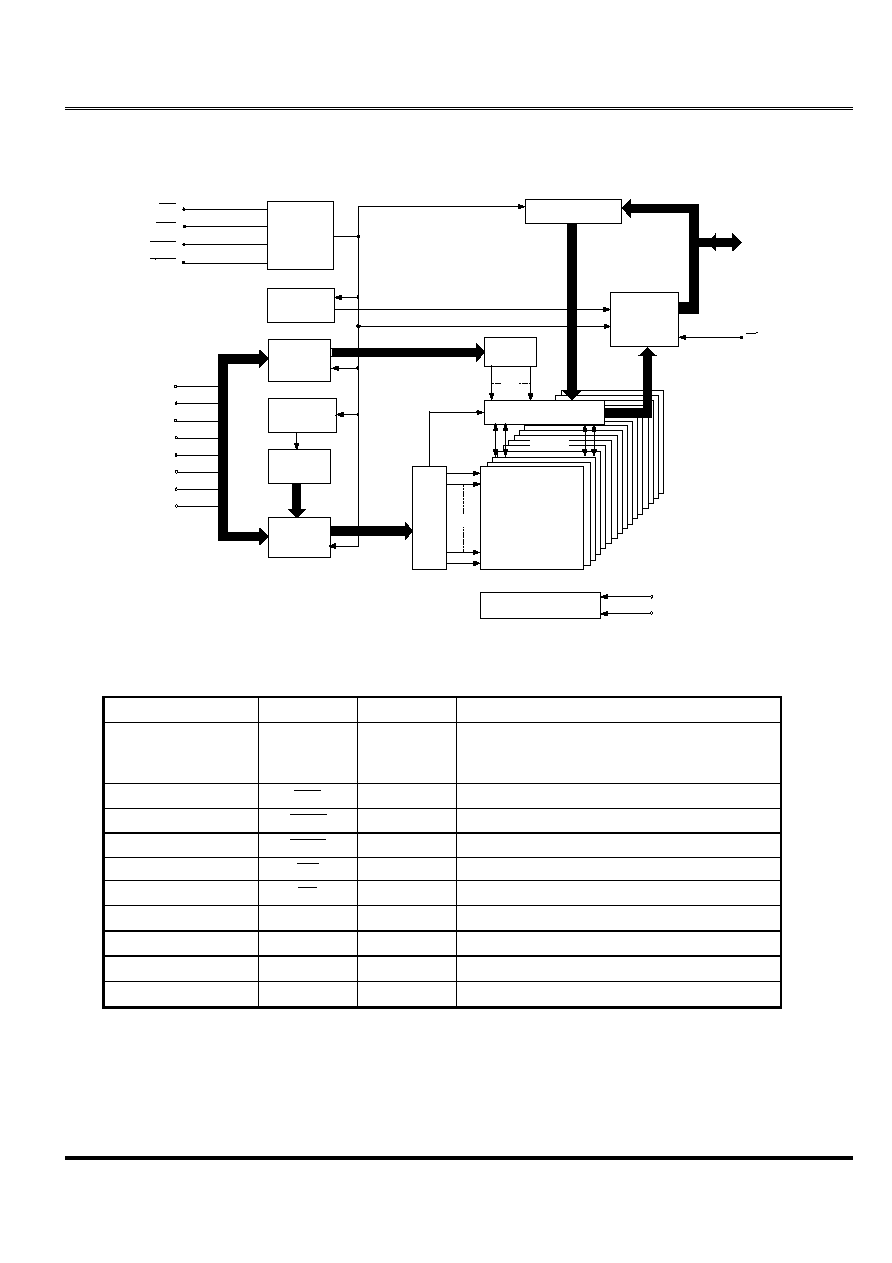(OLWH07
M11B11664A
Elite Memory Technology Inc
Publication Date : Dec. 2000
Revision : 1.3 2/15
FUNCTIONAL BLOCK DIAGRAM
PIN DESCRIPTIONS
PIN NO.
PIN NAME
TYPE
DESCRIPTION
16~19,22~25
A0~A7
Input
14
RAS
Input
28
CASH
Input
Column Address Strobe / Upper Byte Control
29
CASL
Input
Column Address Strobe / Lower Byte Control
13
WE
Input
Write Enable
27
OE
Input
Output Enable
2~5,7~10,31~34,36~39
I/O0 ~ I/O15
Input / Output Data Input / Output
1,6,20
V
CC
Supply
Power, 5V
21,35,40
V
SS
Ground
Ground
11,12,15,30
NC
-
No Connect
CONTROL
LOGIC
DATA-IN BUFFER
CLOCK
GENERATOR
DATA-OUT
BUFFER
COLUMN
BUFFER
REFRESH
CONTROLER
REFRESH
COUNTER
ROW.
BUFFERS(8)
9
A0
A1
A2
A3
A4
A5
A6
A7
COLUMN
DECODER
OE
16

R
O
W
DE
CO
DE
R
256 x 256 x 16
MEMORY
ARRAY
16
SENSE AMPLIFIERS
I/O GATING
8
256 x 16
V
CC
V
SS
IO0
:
IO15
RAS
CASH
256
256
8
8
8
8
8
CASL
V
BB
GENERATOR
WE
16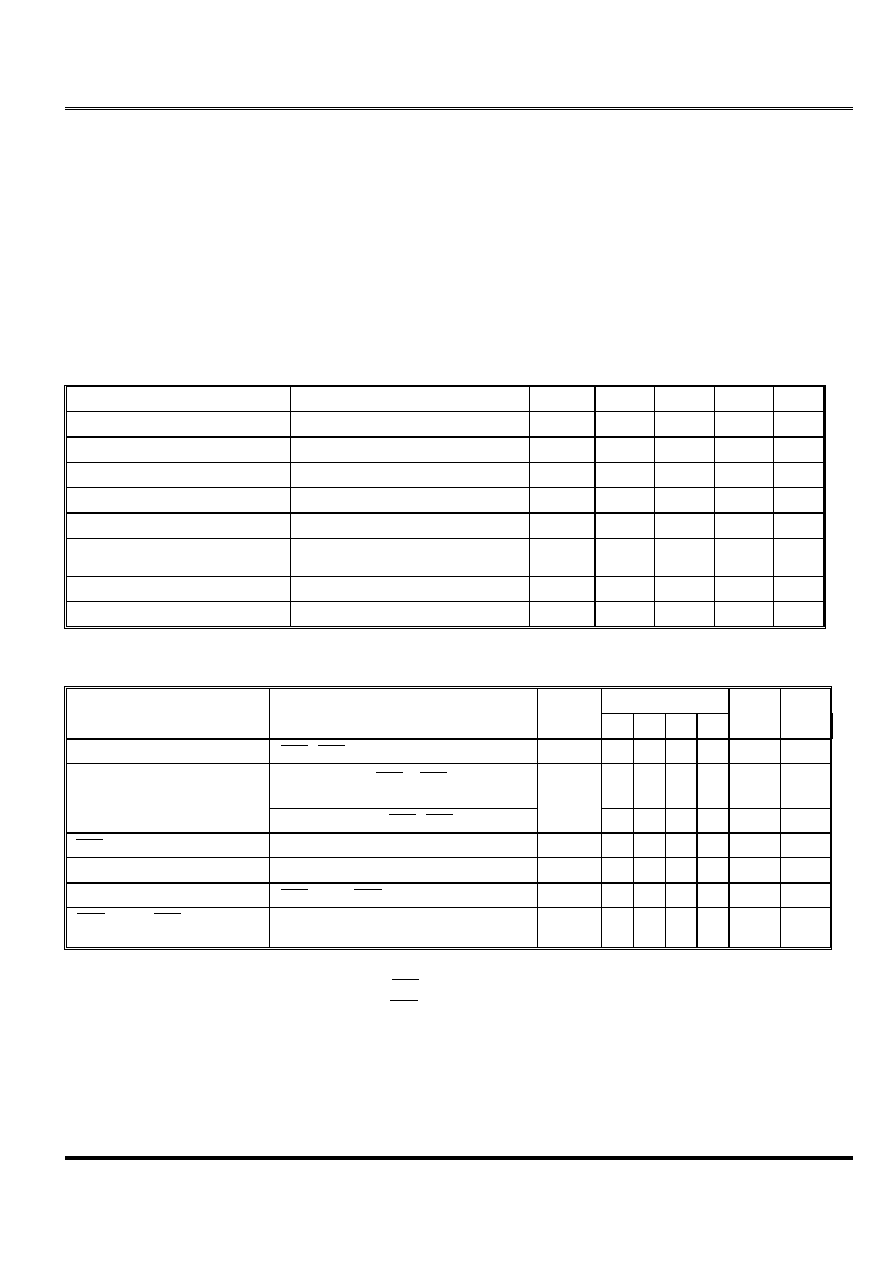(OLWH07
M11B11664A
Elite Memory Technology Inc
Publication Date : Dec. 2000
Revision : 1.3 3/15
ABSOLUTE MAXIMUM RATINGS
Voltage on Any pin Relative to Vss ... ......-1V to +7V
Operating Temperature, T
A
(ambient) ....0 C
to +70 C
Storage Temperature (plastic) ..........-55 C
to +150 C
Power Dissipation .......................................1.0W
Short Circuit Output Current ........................50mA
Permanent device damage may occur if "Absolute
Maximum Ratings" are exceeded. This is a stress rating
only, and functional operation of the device above those
conditions indicated in the operational sections of this
specification is not implied. Exposure to absolute
maximum rating conditions for extended periods may
affect reliability.
DC ELECTRICAL CHARACTERISTICS AND RECOMMENDED
OPERATING CONDITIONS
(0 C
T
A
70 C
; V
CC
= 5V
10% unless otherwise noted)
PARAMETER
CONDITIONS
SYMBOL
MIN
MAX
UNITS NOTES
Supply Voltage
V
CC
4.5
5.5
V
1
Supply Voltage
V
SS
0
0
V
Input High Voltage
V
IH
2.4
V
CC
+1
V
1
Input Low Voltage
V
IL
-1.0
0.8
V
1
Input Leakage Current
0V
V
IH
7V
I
LI
-10
10
A
Output Leakage Current
0V
V
OUT
7V
Output(s) disable
I
LO
-10
10
A
Output High Voltage
I
OH
= -5 mA
V
OH
2.4
-
V
Output Low Voltage
I
OL
= 4.2 mA
V
OL
-
0.4
V
Note : 1.All Voltages referenced to V
SS
MAX
UNITS NOTES
PARAMETER
CONDITIONS
SYMBOL
-25 -30 -35 -40
Operating Current
RAS
, CAS cycling , t
RC
=min
I
CC1
170 150 130 120
mA
1,2
TTL interface , RAS , CAS = V
IH
,
D
OUT
=High-Z
4
4
4
4
mA
Standby Current
CMOS interface, RAS , CAS
V
CC
-0.2V
I
CC2
2
2
2
2
mA
RAS
only refresh Current
t
RC
= min
I
CC3
170 150 130 120
mA
2
EDO Page Mode Current
t
PC
= min
I
CC4
170 150 130 120
mA
1,3
Standby Current
RAS
=V
IH
, CAS = V
IL
I
CC5
5
5
5
5
mA
1
CAS
Before RAS Refresh
Current
t
RC
= min
I
CC6
170 150 130 120
mA
Note : 1. ICC max is specified at the output open condition.
2. Address can be changed twice or less while RAS =V
IL .
3. Address can be changed once or less while CAS =V
IH
.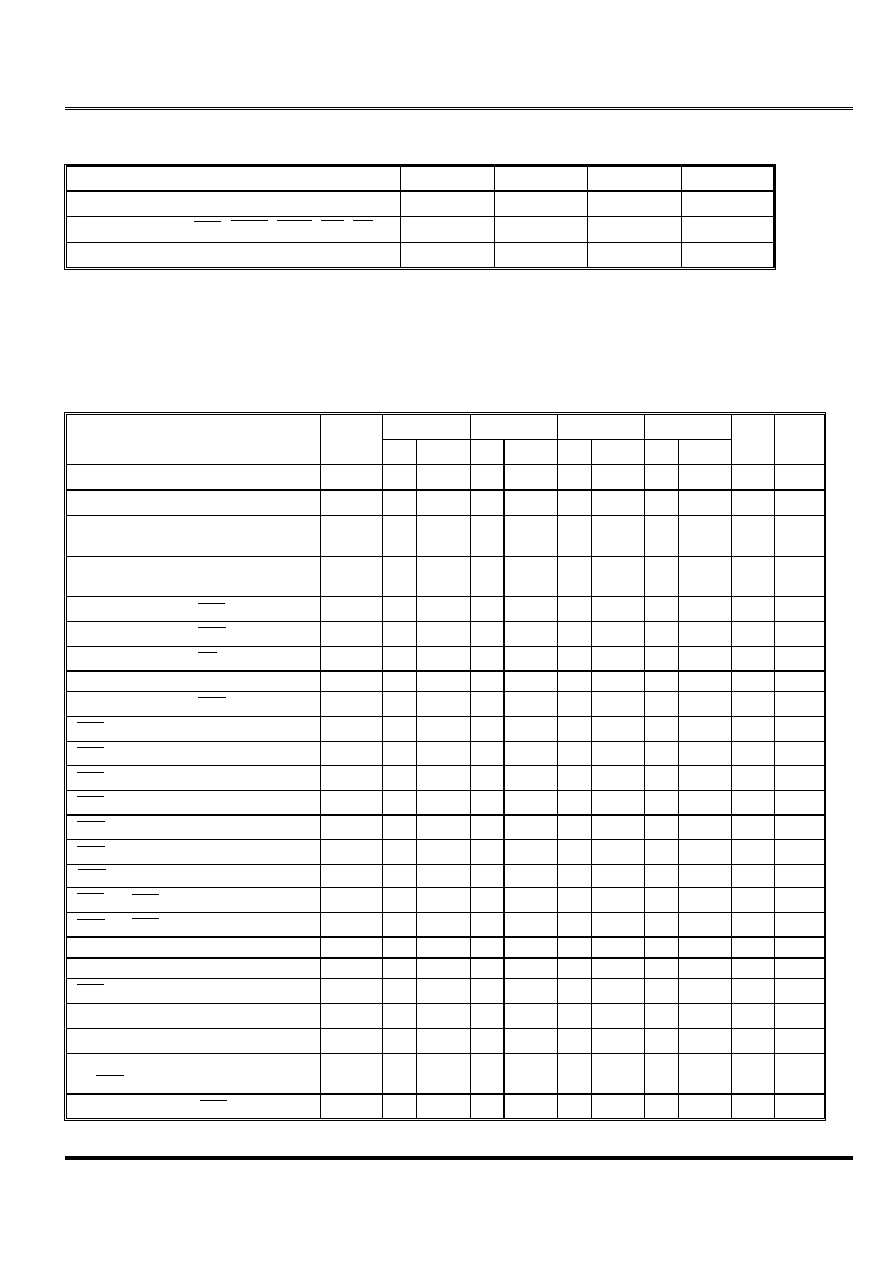(OLWH07
M11B11664A
Elite Memory Technology Inc
Publication Date : Dec. 2000
Revision : 1.3 4/15
CAPACITANCE
(Ta = 25 C
, V
CC
= 5V
10%)
PARAMETER
SYMBOL
TYP
MAX
UNIT
C
I1
-
5
pF
Input Capacitance ( RAS , CASH , CASL , WE , OE )
C
I2
-
7
pF
Output capacitance (I/O0~I/O15)
C
I / O
-
10
pF
AC ELECTRICAL CHARACTERISTICS
(Ta = 0 to 70 C
, V
CC
=5V
10%, V
SS
= 0V) (note 14)
Test Conditions
Input timing reference levels : 0V, 3V
Output reference level : V
OL
= 0.8V, V
OH
=2.0V
Output Load : 2TTL gate + CL (50pF)
Assumed t
T
= 2ns
-25
-30
-35
-40
PARAMETER
SYMBOL
MIN
MAX
MIN
MAX
MIN
MAX
MIN
MAX
UNIT Notes
t
RC
43
55
65
75
ns
t
RWC
65
85
95
105
ns
Time
t
PC
10
12
14
16
ns
22
Time
t
PCM
32
37
42
47
ns
22
Access Time From RAS
t
RAC
25
30
35
40
ns
4
Access Time From CAS
t
CAC
8
9
10
11
ns
5,20
Access Time From OE
t
OAC
8
9
10
11
ns
13,20
t
AA
12
15
18
20
ns
Access Time From CAS Precharge
t
ACP
14
17
20
22
ns
20
RAS
Pulse Width
t
RAS
25
10,000
30
10,000
35
10,000
40
10,000
ns
RAS
Pulse Width (EDO Page Mode)
t
RASC
25 100,000 30 100,000 35 100,000 40 100,000
ns
RAS
Hold Time
t
RSH
8
9
10
11
ns
25
RAS
Precharge Time
t
RP
15
20
25
30
ns
CAS
Pulse Width
t
CAS
4
10,000
5
10,000
5
10,000
6
10,000
ns
24
CAS
Hold Time
t
CSH
21
26
30
35
ns
19
CAS
Precharge Time
t
CP
4
4
5
5
ns
6,23
RAS
to CAS Delay Time
t
RCD
10
17
10
21
10
25
10
29
ns
7,18
CAS
to RAS Precharge Time
t
CRP
5
5
5
5
ns
19
t
ASR
0
0
0
0
ns
t
RAH
5
5
5
5
ns
RAS
t
8
13
8
15
8
17
8
20
ns
8
t
ASC
0
0
0
0
ns
18
t
CAH
5
5
5
5
ns
18
to RAS )
t
AR
22
26
30
34
ns
t
RAL
12
15
18
20
ns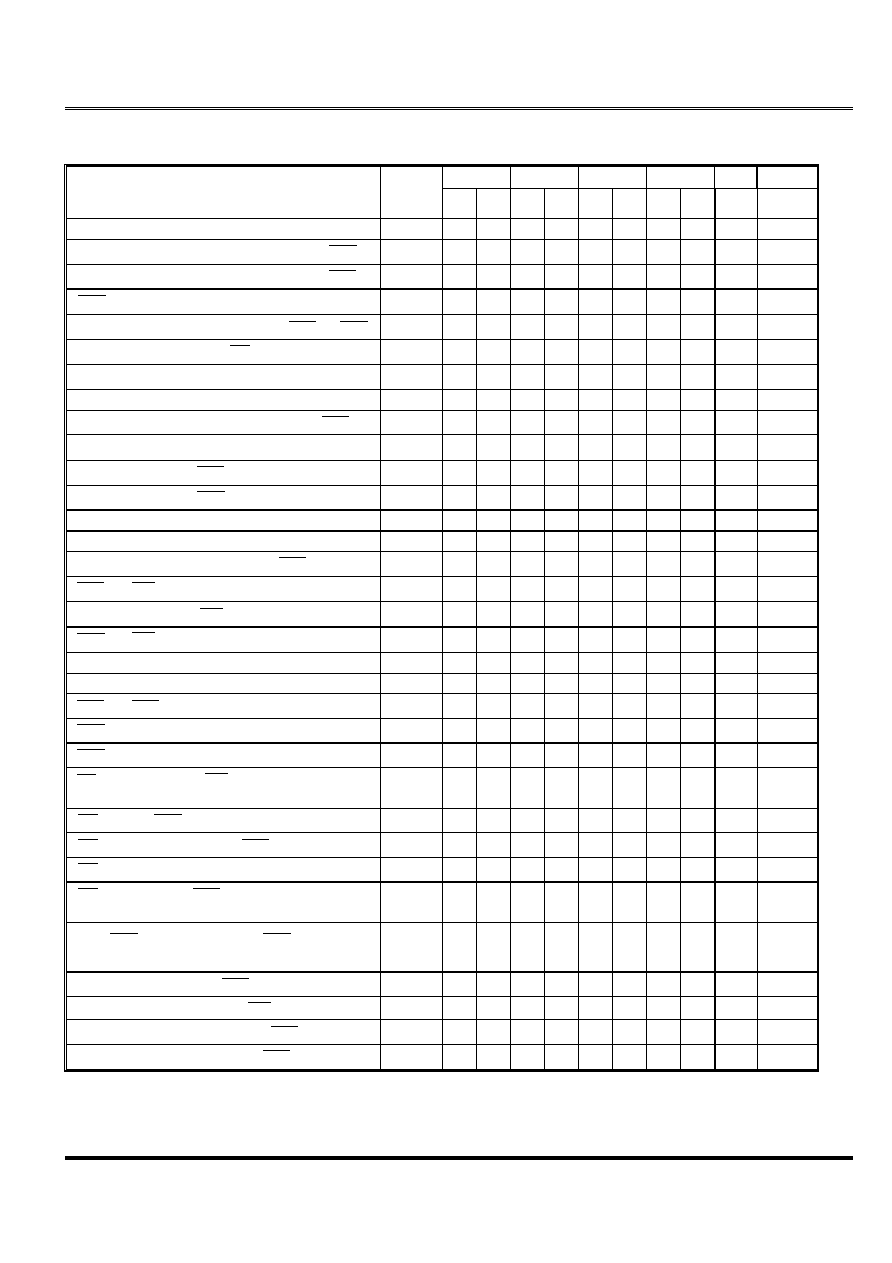(OLWH07
M11B11664A
Elite Memory Technology Inc
Publication Date : Dec. 2000
Revision : 1.3 5/15
(Continued)
-25
-30
-35
-40
UNIT
Notes
PARAMETER
SYMBOL
MIN MAX MIN MAX MIN MAX MIN MAX
t
RCS
0
0
0
0
ns
15,18
Read Command Hold Time Reference to CAS
t
RCH
0
0
0
0
ns
9,15,19
Read Command Hold Time Reference to RAS
t
RRH
0
0
0
0
ns
9
CAS
to Output in Low-Z
t
CLZ
3
3
3
3
ns
20
Output Buffer Turn-off Delay From CAS or RAS
t
OFF1
3
15
3
15
3
15
3
15
ns
10,17,20
Output Buffer Turn-off to OE
t
OFF2
6
8
8
8
ns
17,26
Write Command Setup Time
t
WCS
0
0
0
0
ns
11,15,18
Write Command Hold Time
t
WCH
5
5
5
5
ns
15,25
Write Command Hold Time(Reference to RAS )
t
WCR
22
26
30
34
ns
15
Write Command Pulse Width
t
WP
5
5
5
5
ns
15
Write Command to RAS Lead Time
t
RWL
7
8
9
10
ns
15
Write Command to CAS Lead Time
t
CWL
5
6
7
8
ns
15,19
Data-in Setup Time
t
DS
0
0
0
0
ns
12,20
Data-in Hold Time
t
DH
5
5
5
5
ns
12,20
Data-in Hold Time (Reference to RAS )
t
DHR
22
26
30
34
ns
RAS
to WE Delay Time
t
RWD
34
46
51
56
ns
11
Column Address to WE Delay Time
t
AWD
21
31
34
36
ns
11
CAS
to WE Delay Time
t
CWD
17
25
26
27
ns
11,18
Transition Time (rise or fall)
t
T
1.5
50
1.5
50
2.5
50
2.5
50
ns
2,3
Refresh Period (256 cycles)
t
REF
4
4
4
4
ms
RAS
to CAS Precharge Time
t
RPC
10
10
10
10
ns
CAS
Setup Time(CBR REFRESH)
t
CSR
5
10
10
10
ns
1,18
CAS
Hold Time(CBR REFRESH)
t
CHR
7
10
10
10
ns
1,19
OE
Hold Time From WE During Read-Mode-
Write Cycle
t
OEH
4
4
4
5
ns
16
OE
Low to CAS High Setup Time
t
OES
4
4
4
5
ns
OE
High Hold Time From CAS High
t
OEHC
2
2
2
2
ns
OE
Precharge Time
t
OEP
2
2
2
2
ns
OE
Setup Prior to RAS During Hidden Refresh
Cycle
t
ORD
0
0
0
0
ns
Last CAS Going Low to First CAS Returning
High
t
CLCH
4
5
5
6
ns
21
Data Output Hold After CAS Returning Low
t
COH
3
3
3
3
ns
Output Disable Delay From WE
t
WHZ
3
7
3
7
3
7
3
7
ns
Read Setup Time Reference to RAS in CBR
t
RSR
5
5
5
5
ns
Read Hold Time Reference to RAS in CBR
t
RHR
5
5
5
5
ns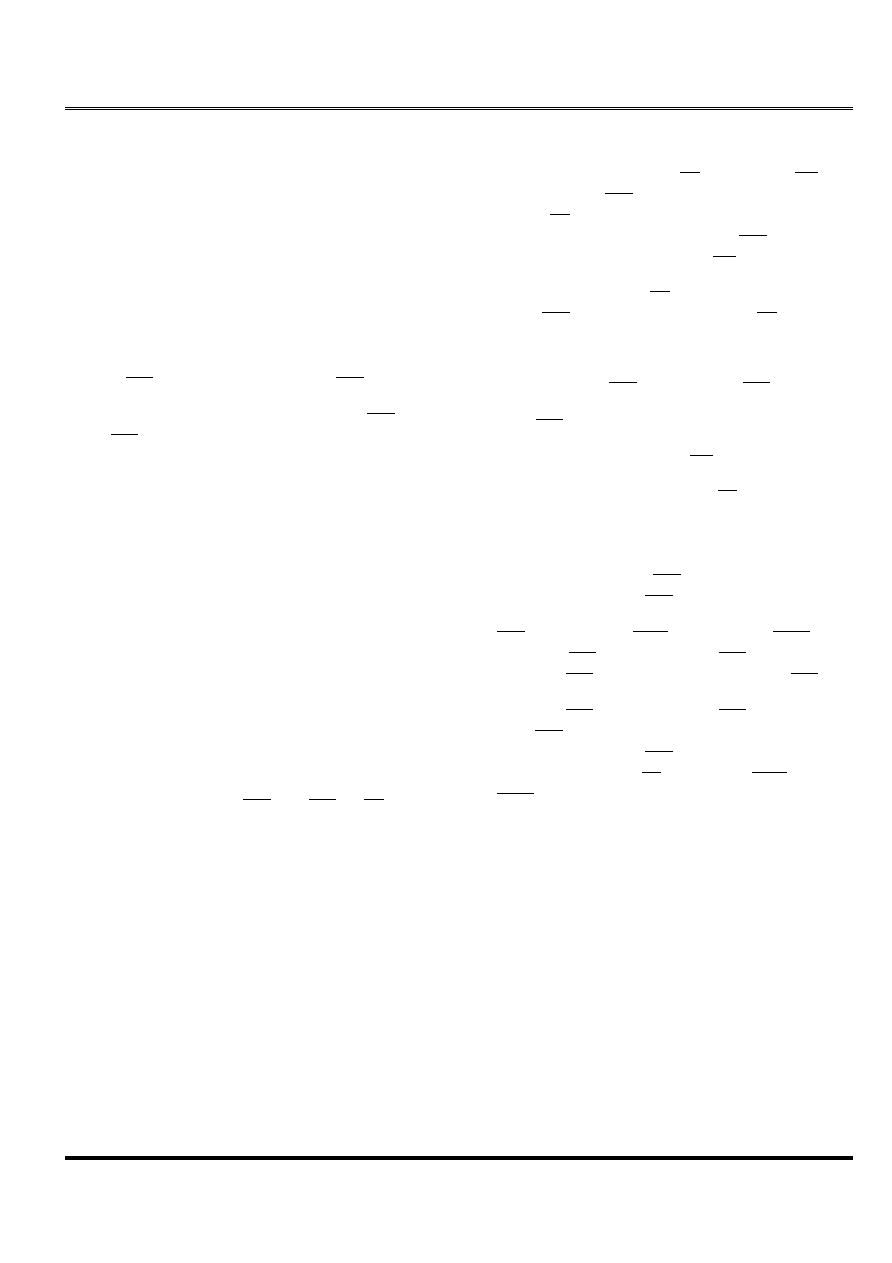(OLWH07
M11B11664A
Elite Memory Technology Inc
Publication Date : Dec. 2000
Revision : 1.3 6/15
Notes :
1.
Enables on-chip refresh and address counters.
2.
V
IH
(min) and V
IL
(max) are reference levels for
measuring timing of input signals. Transition times
are measured between V
IH
and V
IL
.
3.
In addition to meet the transition rate specification, all
input signals must transit between V
IH
and V
IL
in a
monotonic manner.
4.
Assume that t
RCD
< t
RCD
(max). If t
RCD
is greater than
the maximum recommended value shown in this
table, t
RAC
will increase by the amount that t
RCD
exceeds the value shown.
5.
Assume that t
RCD
t
RCD
(max)
6.
If CAS is low at the falling edge of RAS , data-out
will be maintained from the previous cycle. To initiate
a new cycle and clear the data-out buffer, CAS and
RAS
must be pulsed high.
7.
Operation within the t
RCD
limit ensures that t
RCD
(max)
can be met, t
RCD
(max) is specified as a reference
point only ; if t
RCD
is greater than the specified t
RCD
(max) limit, access time is controlled by t
CAC
.
8.
Operation within the t
limit ensures that t
(max)
can be met. t
(max) is specified as a reference
point only ; if t
is greater than the specified t
(max) limit, access time is controlled by t
AA
.
9.
Either t
RCH
or t
RRH
must be satisfied for a READ cycle.
10.
t
OFF1
(max) defines the time at which the output
achieves the open circuit condition ; it is not a
reference to V
OH
or V
OL
.
11.
t
WCS
, t
RWD
, t
AWD
and t
CWD
are restrictive operating
parameters in LATE WRITE and READ-MODIFY-
WRITE cycle only. If t
WCS
t
WCS(min)
, the cycle is an
EARLY WRITE cycle and the data output will remain
an open circuit throughout the entire cycle. If t
RWD
t
RWD(min)
, t
AWD
tAW D(min)
and t
CWD
t
CWD(min)
, the
cycle is READ-WRITE and the data output will
contain data read from the selected cell. If neither of
the above conditions is met, the state of I/O (at
access time and until CAS and RAS or OE go
back to V
IH
) is indeterminate. OE held high and WE
taken low after CAS goes low result in a LATE
WRITE ( OE -controlled) cycle.
12. Those parameters are referenced to CAS leading
edge in EARLY WRITE cycles and WE leading edge
in LATE WRITE or READ-MODIFY- WRITE cycles.
13. During a READ cycle, if OE is low then taken HIGH
before CAS goes high, I/O goes open, if OE is tied
permanently low, a LATE WRITE or READ-MODIFY-
WRITE operation is not possible.
14. An initial pause of 200
s is required after power-up
followed by eight RAS refresh cycles ( RAS only or
CBR) before proper device operation is assured. The
eight RAS cycle wake-ups should be repeated any
time the t
REF
refresh requirement is exceeded.
15. WRITE command is defined as WE going low.
16. LATE WRITE and READ-MODIFY-WRITE cycles must
have both tOFF2 and t
OEH
met ( OE high during
WRITE cycle) in order to ensure that the output buffers
will be open during the WRITE cycles.
17. The I/Os open during READ cycles once t
OFF1
or t
OFF2
occur.
18. Referenced to the earlier CAS falling edge.
19. Referenced to the latter CAS rising edge.
20. Output parameter (I/O) is referenced to corresponding
CAS
input, IO0~7 by CASL and IO8~15 by CASH .
21. Last falling CAS edge to first rising CAS edge.
22. Last rising CAS edge to next cycle's last rising CAS
edge.
23. Last rising CAS edge to first falling CAS edge.
24. Each CAS must meet minimum pulse width.
25. Referenced to the latter CAS failing edge.
26. All IOs controlled by OE , regardless CASL and
CASH
.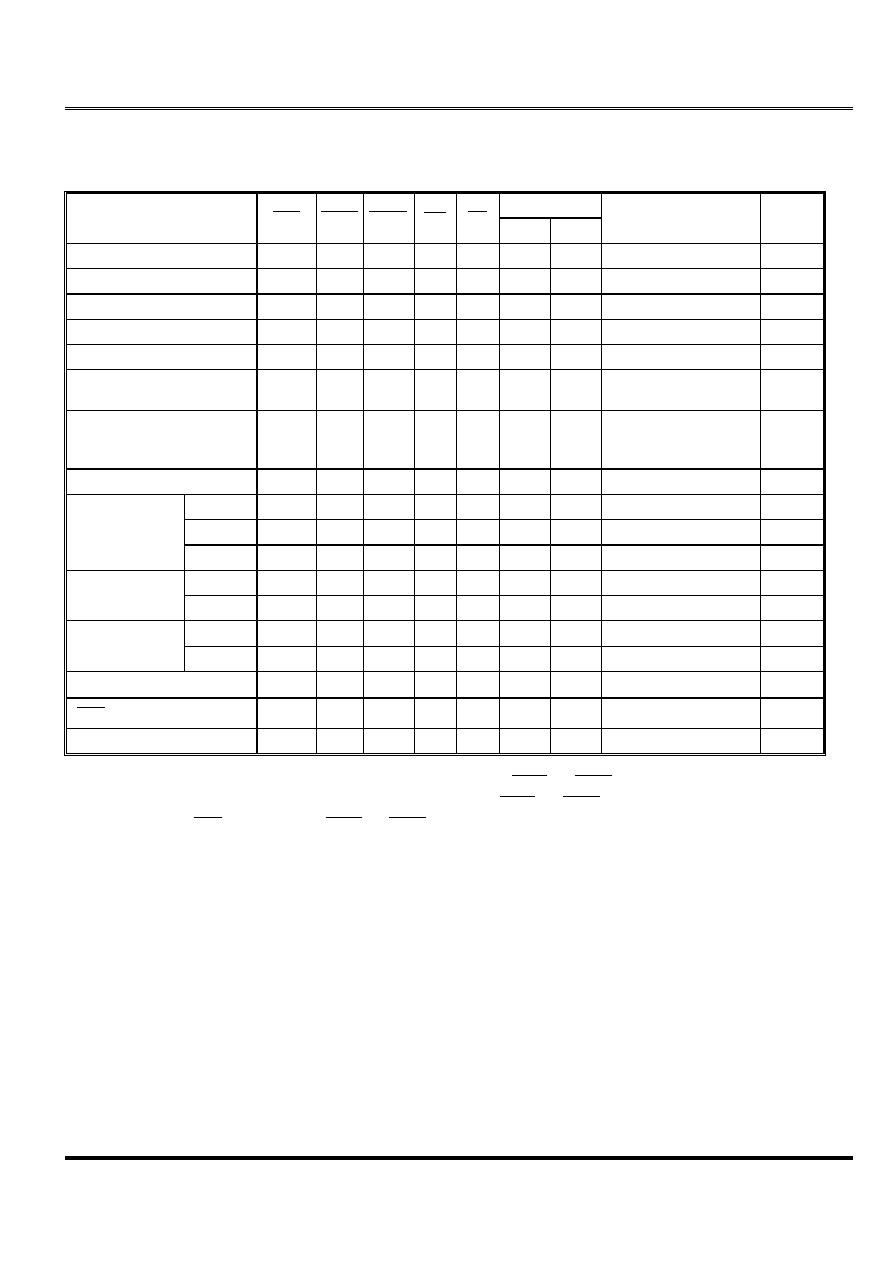(OLWH07
M11B11664A
Elite Memory Technology Inc
Publication Date : Dec. 2000
Revision : 1.3 7/15
TRUTH TABLE
FUNCTION
RAS
CASL CASH
WE
OE
ROW
COL
DQ
S
NOTES
Standby
H
H
X
H
X
X
X
X
X
High-Z
L
L
L
H
L
ROW
COL
Data-Out
L
L
H
H
L
ROW
COL
Lower Byte, Data-Out
L
H
L
H
L
ROW
COL
Upper Byte, Data-Out
Write : Word (Early Write)
L
L
L
L
X
ROW
COL
Data-In
Write : Lower Byte (Early)
L
L
H
L
X
ROW
COL
Lower Byte, Data-In ,
Upper Byte, High-Z
Write : Upper Byte (Early)
L
H
L
L
X
ROW
COL
Lower Byte, High-Z ,
Upper Byte, Data-In
L
L
L
H
L L
H
ROW
COL
Data-Out, Data-In
1, 2
1st Cycle
L
H
L
H
L
H
L
ROW
COL
Data-Out
2
2nd Cycle
L
H
L
H
L
H
L
COL
Data-Out
2
EDO-Page-Mode
Any Cycle
L
L
H
L
H
H
L
Data-Out
2
1st Cycle
L
H
L
H
L
L
X
ROW
COL
Data-In
1
EDO-Page-Mode
Write
2nd Cycle
L
H
L
H
L
L
X
COL
Data-In
1
1st Cycle
L
H
L
H
L
H
L L
H
ROW
COL
Data-Out, Data-In
1, 2
EDO-Page-Mode
2nd Cycle
L
H
L
H
L
H
L L
H
COL
Data-Out, Data-In
1, 2
Hidden Refresh
L
H
L
L
L
H
L
ROW
COL
Data-Out
2
RAS
-Only Refresh
L
H
H
X
X
ROW
High-Z
CBR Refresh
H
L
L
L
H
X
X
X
High-Z
3
*Note : 1. These WRITE cycles may also be BYTE WRITE cycles (either CASL or CASH active).
2. These READ cycles may also be BYTE READ cycles (either CASL or CASH active).
3. Only one CAS must be active ( CASL or CASH ).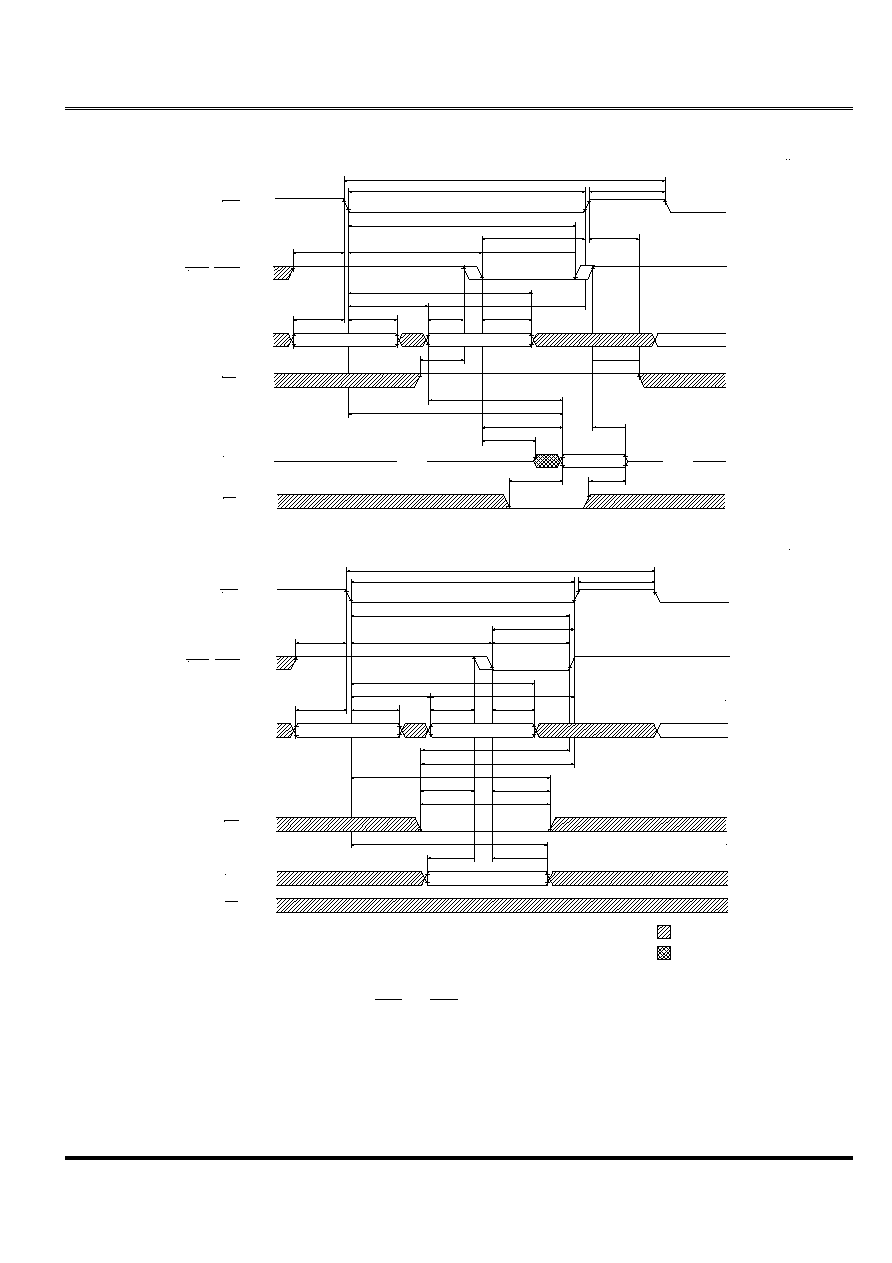(OLWH07
M11B11664A
Elite Memory Technology Inc
Publication Date : Dec. 2000
Revision : 1.3 8/15
Note: 1. t
OFF1
is referenced from the rising edge of RAS or CAS , whichever occurs last.
R A S
V
I H
V
I L
C A S L , C A S H
V
I H
V
I L
V
I H
V
I L
A D D R
V
I H
V
I L
W E
V
O H
V
O L
I / O
V
I H
V
I L
O E
t
R C
t
R A S
t
R P
R O W
C O L U M N
V A L I D D A T A
R O W
t
C R P
t
C S H
t
R S H
t
C A S ,
t
C L C H
t
R R H
O P E N
O P E N
t
O F F 2
t
O A C
t
C L Z
t
C A C
t
R C S
t
R A C
t
A A
t
O F F 1
N O T E 1
t
A S R
t
R A H
t
A S C
t
C A H
t
R A D
t
R A L
t
A R
t
R C D
t
R C H
V A L I D D A T A
R A S
C A S L , C A S H
A D D R
t
R C
t
R A S
t
R P
R O W
C O L U M N
R O W
t
C R P
t
C S H
t
R S H
t
C W L
t
W P
t
W C H
t
A S R
t
R A H
t
A S C
t
C A H
t
R A D
t
R A L
t
A R
t
R C D
t
R W L
t
W C R
t
W C S
t
D H
t
D S
t
D H R
W E
O E
DON'T CARE
UNDEFINED
V
I H
V
I L
V
I H
V
I L
V
I H
V
I L
V
I H
V
I L
V
I H
V
I L
V
I H
V
I L
I / O
t
C A S ,
t
C L C H
EARLY WRITE CYCLE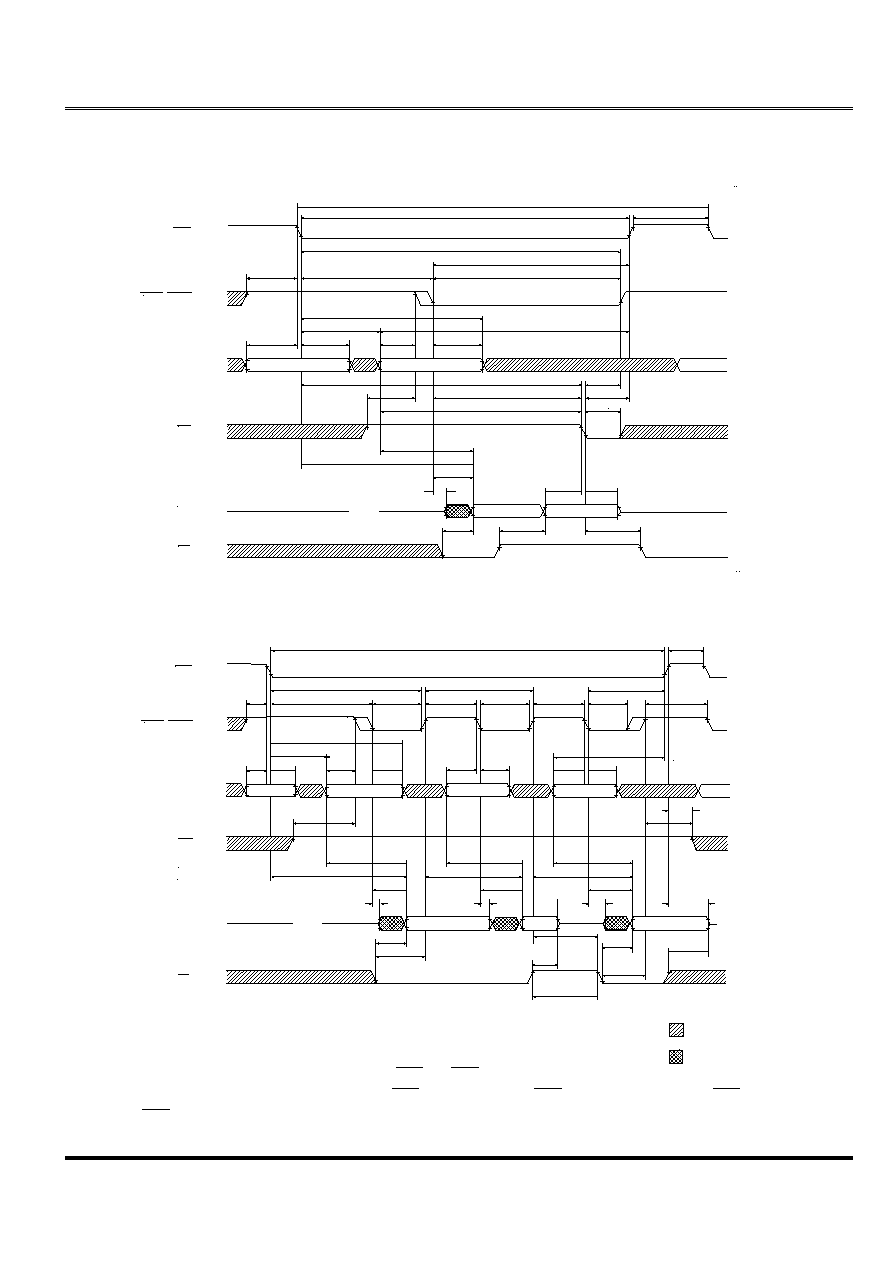(OLWH07
M11B11664A
Elite Memory Technology Inc
Publication Date : Dec. 2000
Revision : 1.3 9/15
*NOTE : 1. t
OFF1
is referenced from the rising edge of RAS or CAS , whichever occurs last.
2. t
PC
can be measured from falling edge of CAS to falling edge of CAS , or from rising edge of CAS to rising edge of
CAS
. Both measurements must meet the t
PC
specification.
R A S
V
I H
V
I L
C A S L , C A S H
V
I H
V
I L
V
I H
V
I L
A D D R
V
I H
V
I L
W E
V
I / O H
V
I / O L
I / O
V
I H
V
I L
O E
t
R W C
t
R A S
t
R P
R O W
C O L U M N
R O W
t
C R P
t
C S H
t
R S H
t
C A S ,
t
C L C H
O P E N
t
D H
t
O F F 2
t
C L Z
t
C A C
t
R C S
t
R A C
t
A A
t
A S R
t
R A H
t
A S C
t
C A H
t
R A D
t
R A L
t
A R
t
R C D
t
C W L
R A S
C A S L , C A S H
A D D R
t
R P
R O W
t
C R P
t
C P
t
C A S ,
t
C L C H
t
R A H
t
A S C
t
C A H
t
R A D
t
A R
t
R C D
W E
O E
DON'T CARE
UNDEFINED
V
I H
V
I L
V
I H
V
I L
V
I H
V
I L
V
I H
V
I L
V
O H
V
O L
V
I H
V
I L
I / O
t
R W L
t
W P
t
A W D
t
R W D
t
C W D
V A L I D D
O U T
t
D S
t
O E H
t
O A C
R O W
t
A S R
t
C A H
t
A S C
t
C A H
t
A S C
t
C S H
C O L U M N
t
C P
t
P C
( N O T E 2 )
C O L U M N
t
R A L
t
C A S ,
t
C L C H
t
R S H
t
C P
C O L U M N
t
R C S
t
R R H
t
R C H
V A L I D D A T A
V A L I D
D A T A
t
C A C
t
C L Z
t
R A C
t
A A
t
C A C
t
C O H
t
A C P
t
A A
t
C A C
t
C L Z
t
A C P
t
A A
V A L I D D A T A
O P E N
N O T E 1
t
O F F 1
O P E N
t
O A C
t
O E S
t
O F F 2
t
O E H C
t
O A C
t
O E S
t
O E P
t
O F F 2
t
R A S C
V A L I D D
I N
t
C A S ,
t
C L C H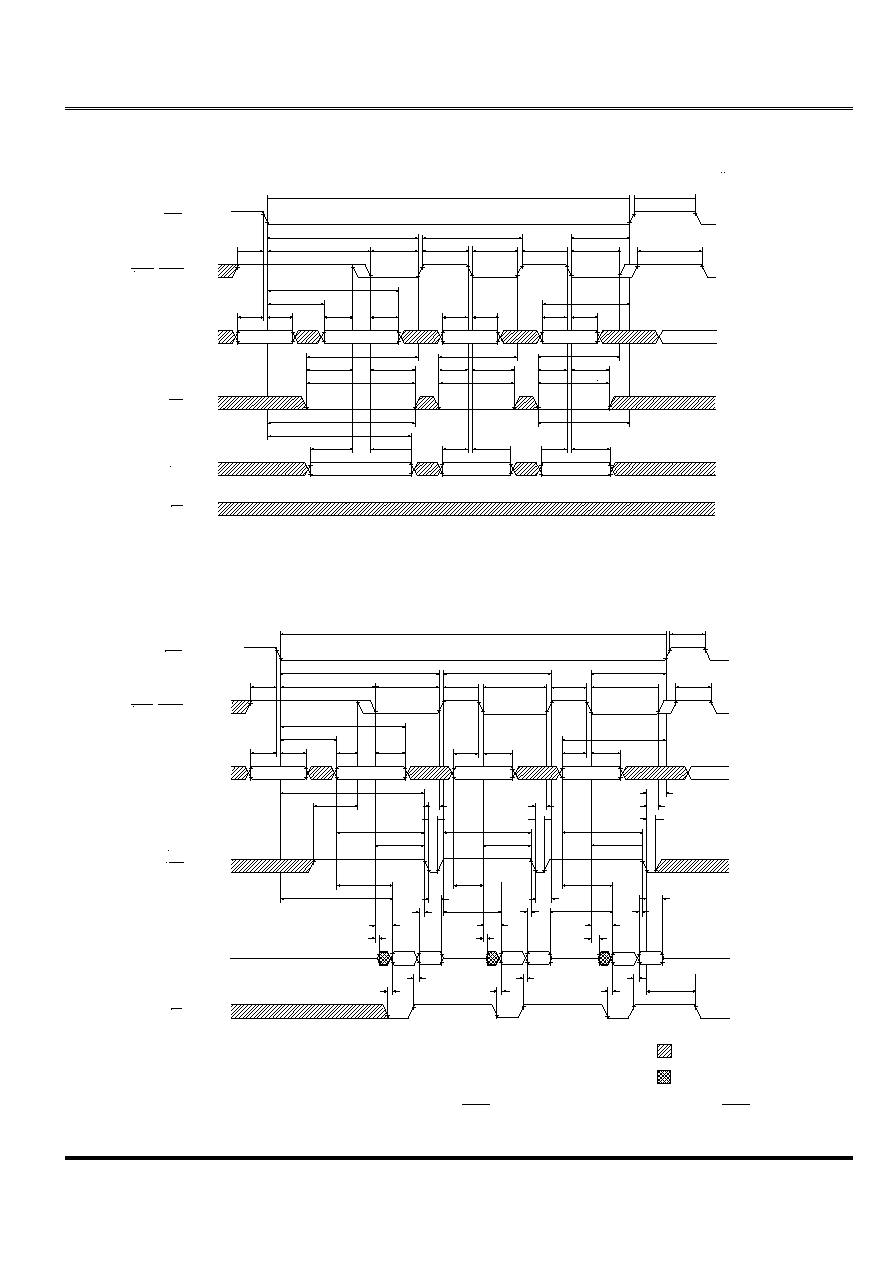(OLWH07
M11B11664A
Elite Memory Technology Inc
Publication Date : Dec. 2000
Revision : 1.3 10/15
EDO-PAGE-MODE EARLY-WRITE CYCLE
Note : 1. t
PC
can be measured from falling edge to falling edge of CAS , or from rising edge to rising edge of CAS . Both
measurements must meet the t
PC
specification.
R A S
V
I H
V
I L
C A S L , C A S H
V
I H
V
I L
V
I H
V
I L
A D D R
V
I H
V
I L
W E
V
I H
V
I L
I / O
V
I H
V
I L
O E
t
R A S C
t
R P
t
C R P
t
D S
t
W C S
t
D H R
t
W C R
t
A S R
t
R A H
t
A S C
t
C A H
t
R A D
t
A R
t
C W L
R A S
C A S L , C A S H
A D D R
W E
OE
DON'T CARE
UNDEFINED
V
I H
V
I L
V
I H
V
I L
V
I H
V
I L
V
I H
V
I L
V
I / O H
V
I / O L
V
I H
V
I L
I / O
t
W C H
t
W P
t
W P
t
C W L
t
W C H
C O L U M N
R O W
t
C P
t
C A S ,
t
C L C H
t
R C D
t
C S H
t
C A S ,
t
C L C H
t
C P
t
P C
( N O T E 1 )
t
C A S ,
t
C L C H
t
C P
t
R S H
t
A S C
t
C A H
C O L U M N
t
A S C
t
C A H
C O L U M N
t
R A L
R O W
t
W C S
t
W P
t
C W L
t
W C H
t
W C S
V A L I D D A T A
t
D H
t
D S
t
D H
t
D S
t
D H
t
R W L
t
R A S C
t
R P
t
C R P
t
R C S
t
A S R
t
R A H
t
A S C
t
C A H
t
R A D
t
A R
t
C W D
t
R W D
t
A W D
t
C A S ,
t
C L C H
t
R C D
t
C S H
t
C A S ,
t
C L C H
t
C P
t
P C M
t
C A S ,
t
C L C H
t
C P
t
R S H
t
A S C
t
C A H
t
A S C
t
C A H
t
R A L
t
C P
R O W
t
C W L
t
W P
t
C W D
t
A W D
t
C W L
t
W P
t
C W D
t
A W D
t
C W L
t
W P
t
R W L
t
C L Z
t
C A C
t
D S
t
D H
V A L I D
D
O U T
V A L I D
D
I N
t
R A C
t
A A
V A L I D
D
O U T
V A L I D
D
I N
t
C L Z
t
C A C
t
D S
t
D H
t
A A
t
A C P
t
A A
t
A C P
t
C L Z
t
C A C
t
D S
t
D H
V A L I D
D
O U T
t
O A C
t
O A C
t
O F F 2
t
O A C
t
O F F 2
t
O E H
V A L I D
D
I N
t
O F F 2
V A L I D D A T A
V A L I D D A T A
C O L U M N
C O L U M N
C O L U M N
R O W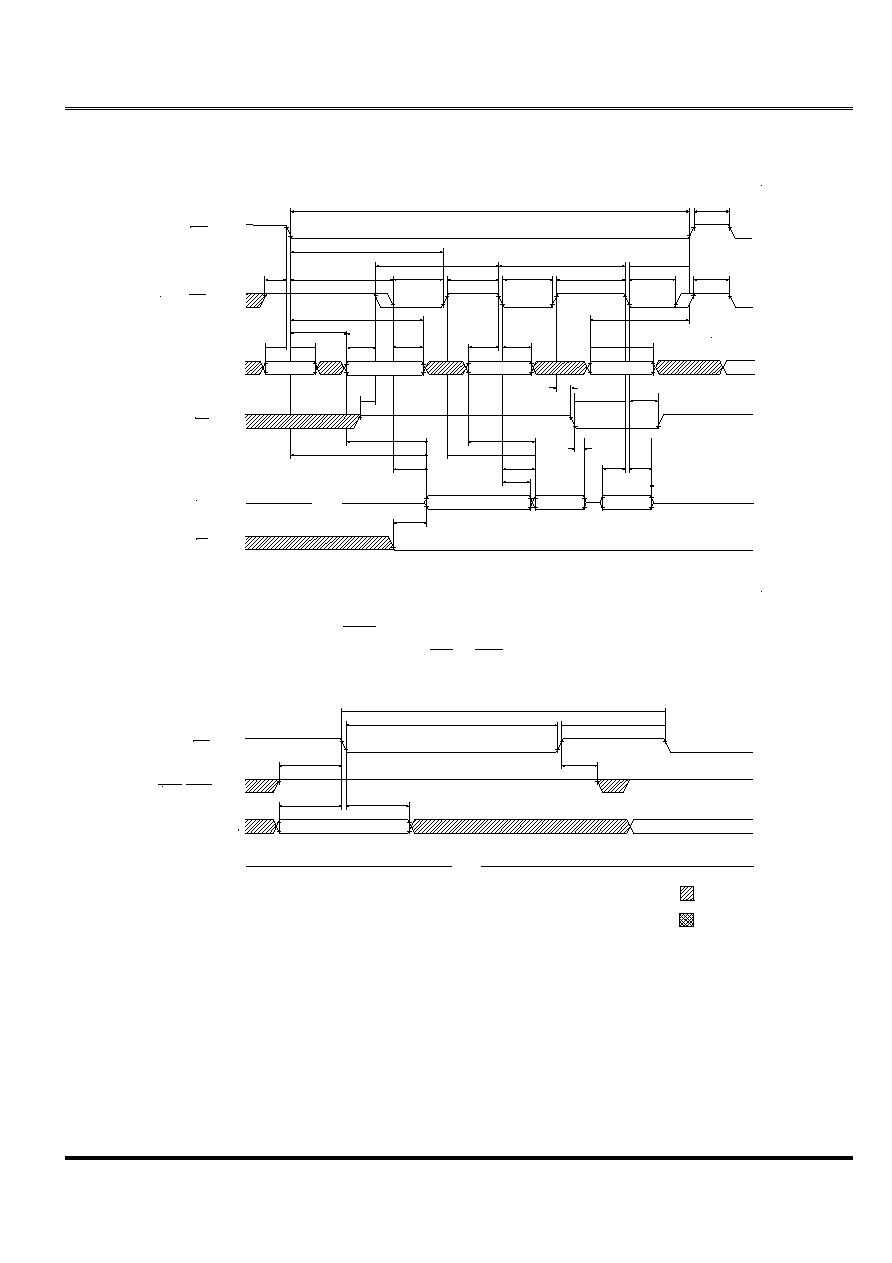(OLWH07
M11B11664A
Elite Memory Technology Inc
Publication Date : Dec. 2000
Revision : 1.3 11/15
R A S
V
I H
V
I L
C A S
V
I H
V
I L
V
I H
V
I L
A D D R
V
I H
V
I L
W E
V
I / O H
V
I / O L
I / O
V
I H
V
I L
O E
R A S
C A S L , C A S H
A D D R
DON'T CARE
UNDEFINED
V
I H
V
I L
V
I H
V
I L
V
I H
V
I L
V
O H
V
O L
I / O
t
R P
R O W
t
C R P
t
C P
t
C A S
t
P C
t
R A H
t
A S C
t
C A H
t
R A D
t
A R
t
R C D
R O W
t
A S R
t
C A H
t
A S C
t
C A H
t
A S C
t
C S H
C O L U M N ( B )
t
C P
t
C A S
t
C P
C O L U M N ( N )
t
R A L
t
C A S
t
C P
C O L U M N ( A )
t
R C S
t
R C H
t
W C S
V A L I D
D A T A ( B )
t
C A C
t
R A C
t
A A
t
C A C
t
A S R
t
A A
t
W H Z
O P E N
t
O A C
t
D S
t
R A S C
t
W C H
V A L I D
D A T A I N
V A L I D D A T A ( A )
t
C O H
t
D H
t
R S H
t
R A S
t
R C
t
R P C
t
C R P
R O W
R O W
t
R A H
O P E N
t
A C P
t
R P
RAS ONLY REFRESH CYCLE
(ADDR = A0~A7 ; OE , WE = DON'T CARE)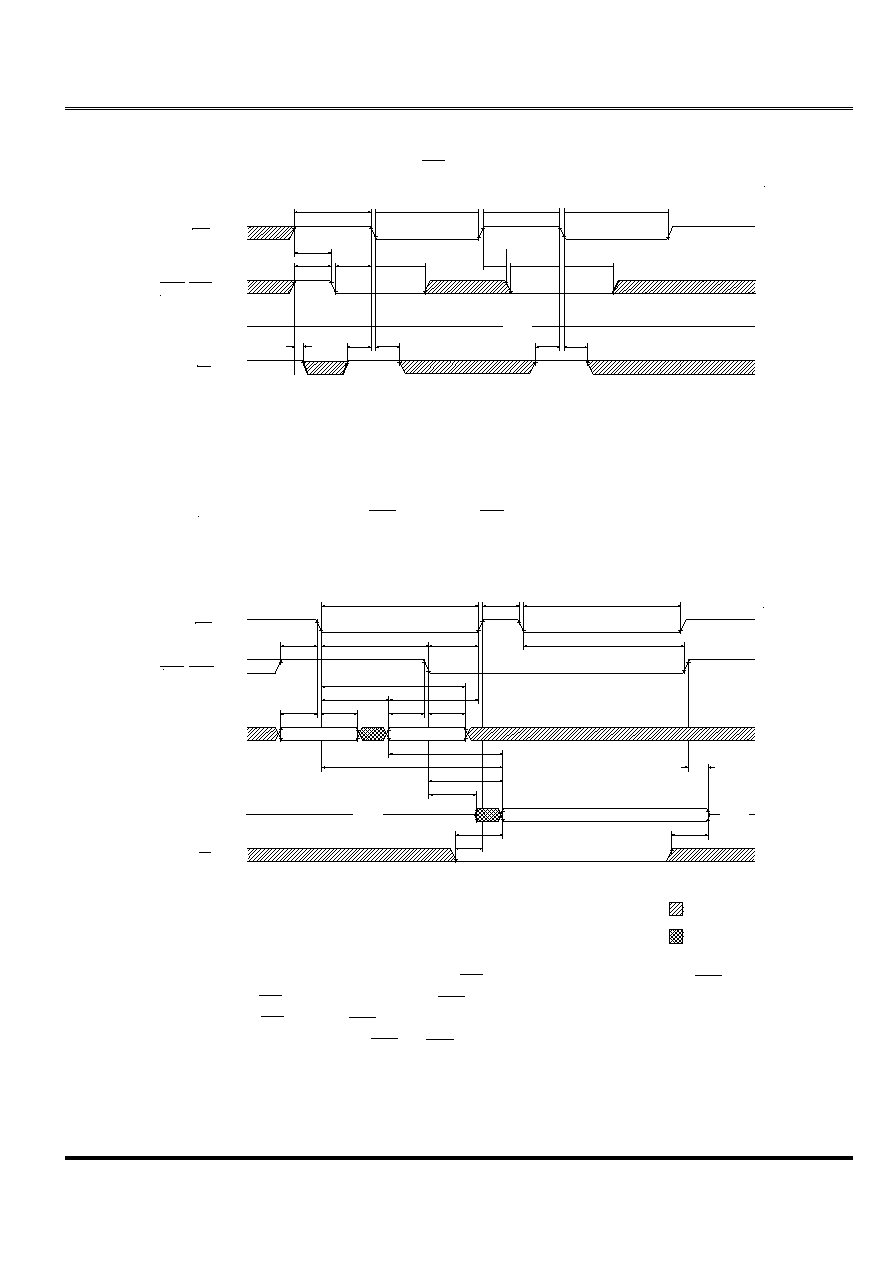(OLWH07
M11B11664A
Elite Memory Technology Inc
Publication Date : Dec. 2000
Revision : 1.3 12/15
CBR REFRESH CYCLE
(A0~A7 ; OE = DON'T CARE)
Note : 1. t
RSR
and t
RHR
are for system design reference only. The WE signal is actually a "don't care" at RAS time during a CBR
REFRESH. However, WE should be held HIGH at RAS time during a CBR REFRESH to ensure compatibility with other
DRAMs which require WE HIGH at RAS time during a CBR REFRESH.
2. t
OFF1
is reference from the rising edge of RAS or CAS , whichever occurs last.
R A S
C A S L , C A S H
A D D R
V
I H
V
I L
V
I H
V
I L
V
I H
V
I L
V
O H
V
O L
I / O
( R E A D )
t
R A S
t
R P
( R E F R E S H )
t
R A S
t
C H R
t
R S H
t
C R P
t
R C D
R O W
C O L U M N
t
A S R
t
R A H
t
A S C
t
R A D
t
C A H
t
R A L
t
A R
V A L I D D A T A
O P E N
O P E N
t
A A
t
R A C
t
C A C
t
C L Z
N O T E 2
t
O F F 1
V
I H
V
I L
O E
t
O A C
t
O R D
t
O F F
2
D O N ' T C A R E
U N D E F I N E D
R A S
V
I H
V
I L
C A S L , C A S H
V
I H
V
I L
V
I H
V
I L
W E
I / O
t
R P C
t
R P
t
C P
t
C S R
t
R S R
t
R H R
t
R S R
t
R H R
t
C S R
t
C H R
t
R P C
t
R P
t
C H R
t
R A S
O P E N
t
R A S
V
O H
V
O L
( N O T E 1 )
t
R C H
HIDDEN REFRESH CYCLE
WE = HIGH ; OE = LOW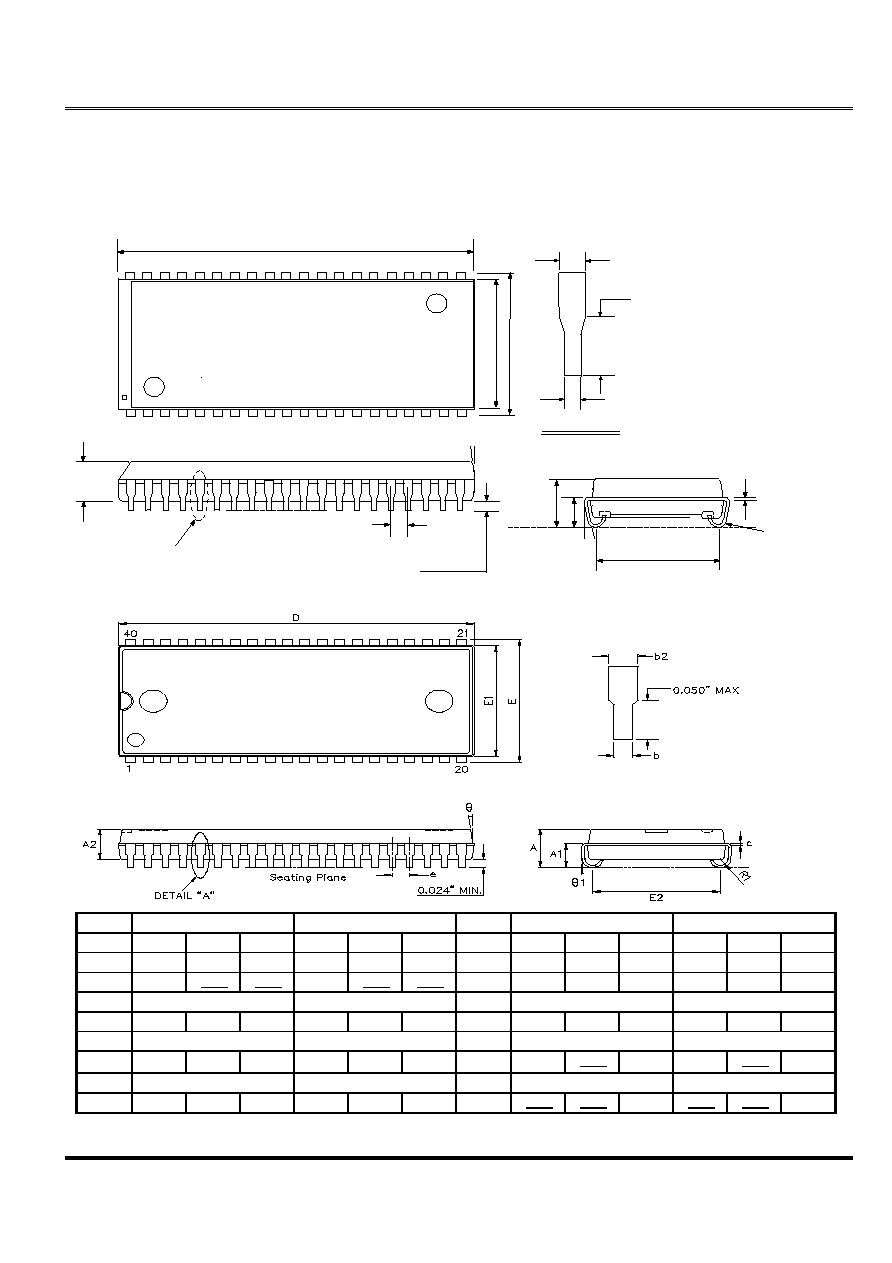(OLWH07
M11B11664A
Elite Memory Technology Inc
Publication Date : Dec. 2000
Revision : 1.3 13/15
E
0\$;
'(7\$,/ \$
'
E
( (
H
'(7\$,/ \$
\$
0,1
\$
F
(
5
\$
;
PACKING DIMENSIONS
SECTIONI
Symbol
Dimension in mm
Dimension in inch
Symbol
Dimension in mm
Dimension in inch
Min
Norm
Max
Min
Norm
Max
Min
Norm
Max
Min
Norm
Max
A
3.250
3.510
3.760
0.128
0.138
0.148
E
10.920 11.176
11.430
0.430
0.440
0.450
A1
2.080
0.082
E1
10.030 10.160 10.290
0.395
0.400
0.405
A2
2.790 REF
0.110 REF
E2
9.40 BSC
0.370 BSC
b
0.380
0.460
0.560
0.015
0.018
0.022
R1
0.760
0.890
1.020
0.030
0.035
0.040
b2
0.635 REF
0.025 REF
b2
0.635 REF
0.025 REF
c
0.180
0.250
0.360
0.007
0.010
0.014
1
e
1.270 BSC
0.050 BSC
e
1.270 BSC
0.050 BSC
D
25.91
26.040 26.290
1.02
1.025
1.035
y1
0.381
0.015
SECTIONII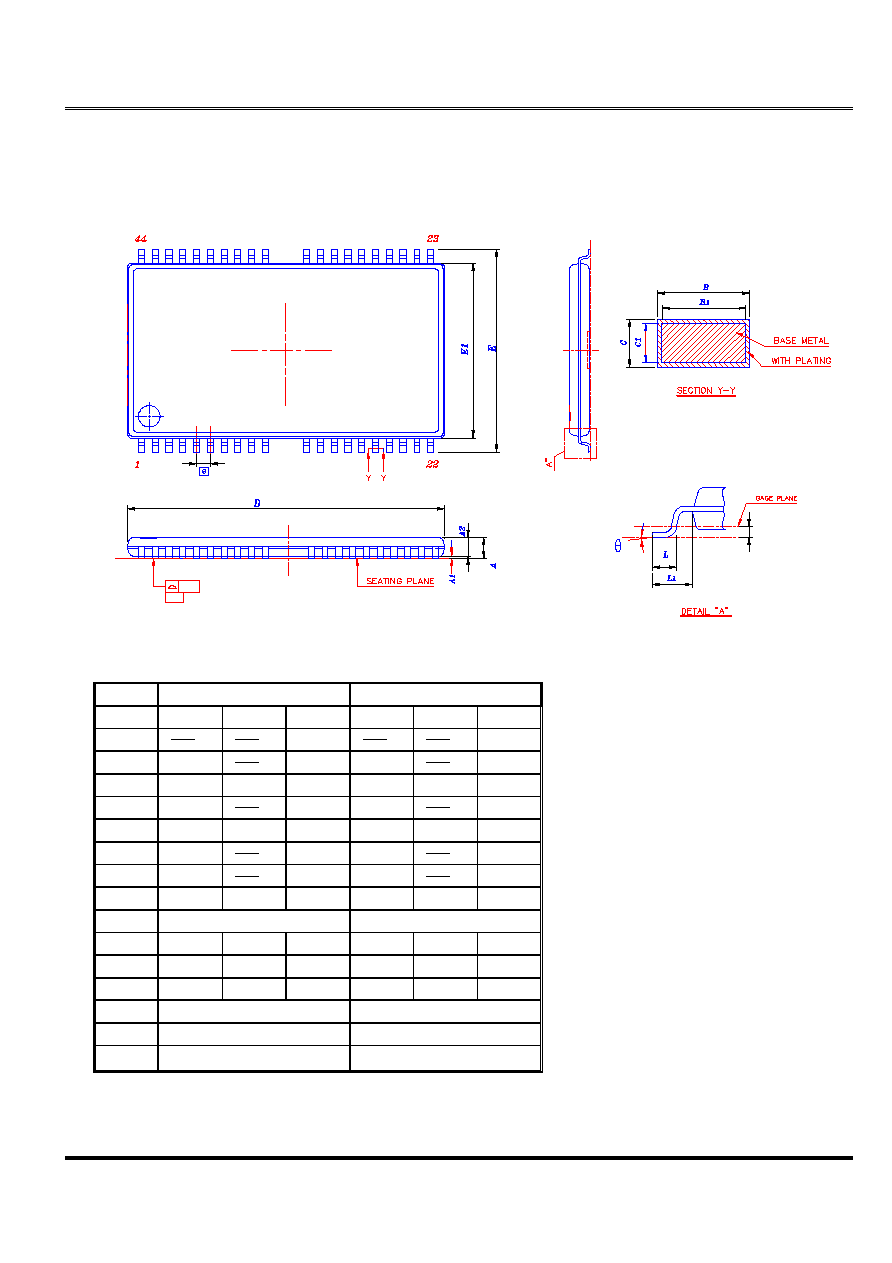(OLWH07
M11B11664A
Elite Memory Technology Inc
Publication Date : Dec. 2000
Revision : 1.3 14/15
PACKING
DIMENSIONS
TSOP(II)
DRAM(400mil)
Symbol
Dimension in mm
Dimension in inch
Min
Norm
Max
Min
Norm
Max
A
1.20
0.047
A1
0.05
0.15
0.002
0.006
A2
0.95
1.00
1.05
0.037
0.039
0.042
b
0.30
0.45
0.012
0.018
b1
0.30
0.35
0.40
0.012
0.014
0.016
c
0.12
0.21
0.005
0.008
c1
0.10
0.16
0.004
0.006
D
18.28
18.41
18.54
0.720
0.725
0.730
ZD
0.805 REF
0.0317 REF
E
11.56
11.76
11.96
0.455
0.463
0.471
E1
10.03
10.16
10.29
0.395
0.400
0.4
L
0.40
0.59
0.69
0.016
0.023
0.027
L1
0.80 REF
0.031 REF
e
0.80 BSC
0.0315 BSC
O ~
7 REF
O ~
7 REF(OLWH07
M11B11664A
Elite Memory Technology Inc
Publication Date : Dec. 2000
Revision : 1.3 15/15
,PSRUWDQW 1RWLFH
\$OO ULJKWV UHVHUYHG
1R SDUW RI WKLV GRFXPHQW PD\ EH UHSURGXFHG RU GXSOLFDWHG LQ DQ\ IRUP RU E\ DQ\
PHDQV ZLWKRXW WKH SULRU SHUPLVVLRQ RI (OLWH07
7KH FRQWHQWV FRQWDLQHG LQ WKLV GRFXPHQW DUH EHOLHYHG WR EH DFFXUDWH DW WKH WLPH RI
SXEOLFDWLRQ (OLWH07 DVVXPHV QR UHVSRQVLELOLW\ IRU DQ\ HUURU LQ WKLV GRFXPHQW DQG
UHVHUYHV WKH ULJKW WR FKDQJH WKH SURGXFWV RU VSHFLILFDWLRQ LQ WKLV GRFXPHQW ZLWKRXW
QRWLFH
7KH LQIRUPDWLRQ FRQWDLQHG KHUHLQ LV SUHVHQWHG RQO\ DV D JXLGH RU H[DPSOHV IRU WKH
DSSOLFDWLRQ RI RXU SURGXFWV 1R UHVSRQVLELOLW\ LV DVVXPHG E\ (OLWH07 IRU DQ\
LQIULQJHPHQW RI SDWHQWV FRS\ULJKWV RU RWKHU LQWHOOHFWXDO SURSHUW\ ULJKWV RI WKLUG
SDUWLHV ZKLFK PD\ UHVXOW IURP LWV XVH 1R OLFHQVH HLWKHU H[SUHVV LPSOLHG RU
RWKHUZLVH LV JUDQWHG XQGHU DQ\ SDWHQWV FRS\ULJKWV RU RWKHU LQWHOOHFWXDO SURSHUW\
ULJKWV RI (OLWH07 RU RWKHUV
\$Q\ VHPLFRQGXFWRU GHYLFHV PD\ KDYH LQKHUHQWO\ D FHUWDLQ UDWH RI IDLOXUH 7R
PLQLPL]H ULVNV DVVRFLDWHG ZLWK FXVWRPHU V DSSOLFDWLRQ DGHTXDWH GHVLJQ DQG RSHUDWLQJ
VDIHJXDUGV DJDLQVW LQMXU\ GDPDJH RU ORVV IURP VXFK IDLOXUH VKRXOG EH SURYLGHG E\
WKH FXVWRPHU ZKHQ PDNLQJ DSSOLFDWLRQ GHVLJQV
(OLWH07 V SURGXFWV DUH QRW DXWKRUL]HG IRU XVH LQ FULWLFDO DSSOLFDWLRQV VXFK DV EXW QRW
OLPLWHG WR OLIH VXSSRUW GHYLFHV RU V\VWHP ZKHUH IDLOXUH RU DEQRUPDO RSHUDWLRQ PD\
GLUHFWO\ DIIHFW KXPDQ OLYHV RU FDXVH SK\VLFDO LQMXU\ RU SURSHUW\ GDPDJH ,I SURGXFWV
GHVFULEHG KHUH DUH WR EH XVHG IRU VXFK NLQGV RI DSSOLFDWLRQ SXUFKDVHU PXVW GR LWV RZQ
TXDOLW\ DVVXUDQFH WHVWLQJ DSSURSULDWH WR VXFK DSSOLFDWLRQV© 2020 • ChipFind• Контакты• Главная страница